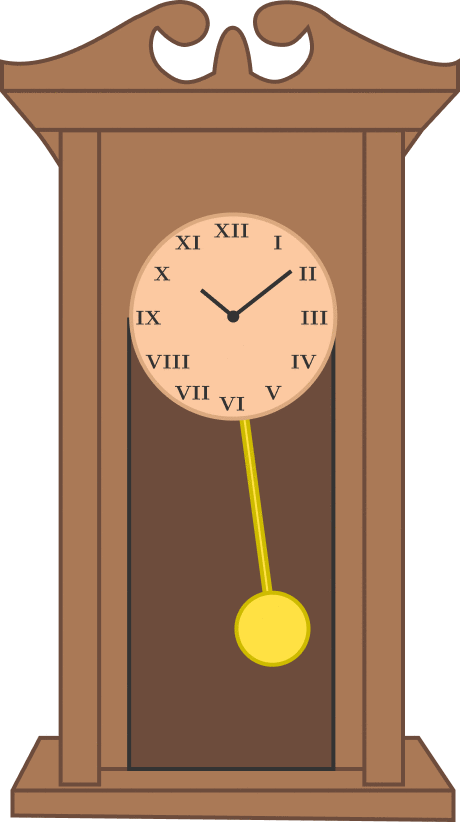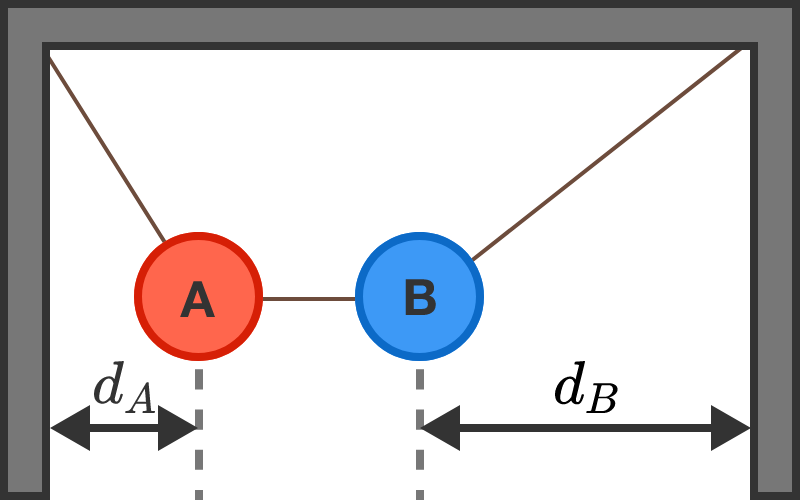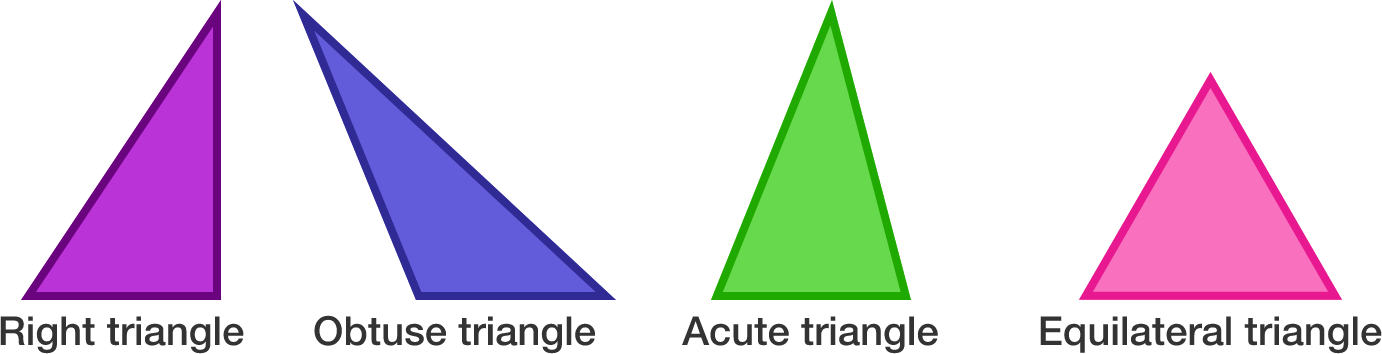# Problems of the Week

Contribute a problem

# 2018-01-29 Basic

A rhombus and a square have the same side length, but are not congruent. Which has the smaller area?A pendulum clock uses the oscillations of a pendulum, a swinging weight, to keep track of time. When the temperature rises and the length of the pendulum increases, what will happen to the clock?

Details and Assumptions

• Ignore the effects of air resistance.As shown in the diagram, spheres A and B are hanging from the corners of the ceiling, and are connected with a string. The centers of spheres A and B are distances $d_A$ and $d_B$ apart from their respective walls.

What can we say about the respective masses $M_A$ and $M_B$ of the spheres based on the distances $d_A$ and $d_B?$

Assume that the system is in equilibrium, that the string joining the masses is taut and horizontal, that the spheres are the same size, and that $d_B>d_A.$

I have chosen four integers ${\color{#D61F06}a}, {\color{#3D99F6}b}, {\color{#20A900}c},$ and ${\color{#69047E}d}$ so that all five of the following sums are values divisible by 3:

• ${\color{#D61F06}a}+{\color{#3D99F6}b}+{\color{#20A900}c}$
• ${\color{#D61F06}a}+{\color{#3D99F6}b}+{\color{#69047E}d}$
• ${\color{#D61F06}a}+{\color{#20A900}c}+{\color{#69047E}d}$
• ${\color{#3D99F6}b}+{\color{#20A900}c}+{\color{#69047E}d}$
• ${\color{#D61F06}a} + {\color{#333333}0}+{\color{#333333}0}.$

Are ${\color{#3D99F6}b},$ ${\color{#20A900}c},$ and ${\color{#69047E}d}$ also guaranteed to be divisible by 3?

The 4 types of triangles below are distinct but have the same area. Which has the smallest perimeter?×